﻿

### On a canonical immersion of the A-jet manifolds into a Grassmann bundle

#### Abstract

For a given smooth manifold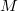$M$ we will consider the ideals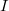$I$ of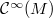$\mathcal{C}^\infty(M)$ such that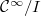$\mathcal{C}^\infty/I$ is a Weil algebra of order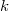$k$; the set of these ideals is the disjoint union of several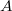$A$-jets manifolds; by fixing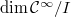$\dim \mathcal{C}^\infty/I$ we will immerse the above mentioned set into a Grassmann bundle of the$k$-th cotangent bundle of$M$, explicitly showing the equations of such an immersion. Finally, in a particular case, we will see how the aforesaid$A$-jets manifolds are placed.

DOI Code: 10.1285/i15900932v20n2p115

Keywords: Jet; Contact element; Weil bundle; Grassmann bundle

Classification: 58A20

Full Text: PDF